## Saturday, 18 June 2011

### Area maximization problem and its variations

First of all, I would like to point out that this is my first time using LaTeX in my blog by iserting image. i don't know why I can't insert LaTeX image by using the official js document. So if you have any ideas plz tell me.

This is a typical question in differentiation topic.
A rectangle is inscribed in an isosceles triangle with base 12 cm and height 10 cm. Find the maximum possible area of the rectangle.

Method 1: Differentiation
This is the most proper way to solve it in secondary level.
Let the height be x cm, then the width is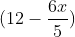, Considering area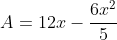, set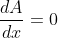, we can get x = 5.
Then A = 30, the maximized area is.

You may notice that the height is as half of the original triangle, so you may wonder are there any other method to deal with this problem...

Method 2: The logic of similar figures
Lemma: When we inscribed similar figures in another consecutively, the ratio of area between the two similar figures are equal.
For example, we have a circle of radius x, now inscribe a square inside, inscribe another circle inside the square and a square in the smaller circle.
Area of Circle 1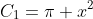Area of Square 1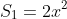Area of Circle 2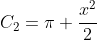Area of Square 2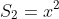Obviously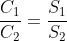The proof is quite simple: they both involve two steps "inscribe square in circle" and "inscribe circle in square". Putting these two steps in different order gives the above result.
Now, you may try to inscribe a triangle in the rectangle, that obviously gives the area ratio of 1/2.
Next, you focus on the area ratio between the large and smaller triangles. The two conditions:
1) Similar figures
2) smaller triangle can be inscribed in the rectangle.
Then you can show that the inscribed triangle is similar to the large one if and only if it's 1/4 of original. Otherwise the dimension of rectangle won't fit the triangle with similar shape.
Therefore, Area ratio of triangle : area of rectangle = 1/2 : 1/4, so area of rectangle = 12*10/4 =Method 3: Area inequlities
Consider the area of triangles (out of the rectangle).
Let the base be k cm, the base of rectangle be (k-x) cm. Then we can show that the sum of areas of triangle is given by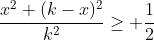Since the inequlities is equivalent to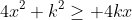which is true by AM-GM inequilities.
Equility holds when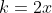, x = 6, then the base of rectangle is half of the triangle, which gives similar result of above.

Method 4: The concept of elasticity
If you study economics, you must heard of the concept of elasticity, and one of its function is to measure the total revenue obtained (PQ) along the demand curve.
Elasticity = % change in P / % change in Q.
Now we are going to give the mathematical frame of this tool.
P is on the y-axis, Q is on the x-axis, % change refers to dx/x
Then we define the elasticity of a curve on the cartesian plane given by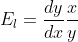.
Focus on the first quadrant. Consider the following fact:
1) Economical fact: Profit will not maximized infinitely by increasing/decreasing the price.
Mathematical fact: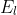changes along a stright line since x,y value varies.
2) Economial fact: Elasticity determine the gain and lose of TR. When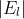<1, a raise in price rise TR. When>1, a rise in price decreases TR. In= 1, the change in price does not change TR.
Mathematical proof: We sayis the ratio of gain and lose in area enclosed due to a infinitely small change in y value. If needs more technique of partial differentiation so we won't discuss that here.
3) Economical fact: TR maximizes as the equilibrium stays at mid-point of the demand curve.
Mathematical proof: For a stright line, we are able to show that the part below and above the mid-point is elastic and inelastic respectively. Then staying in the mid-point maixmizes the area enclosed.
4) Economical fact: the elasticity of curve at mid-point is 1.
Mathematical proof: Consider a line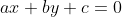. (a,b>0, c<0 so that it passes the first quadrant), then the mid-point between the intercepts is given by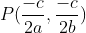while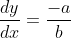, putting them intogives 1.

Combining the above result, we can show that when the height of rectangle is half of that triangle, the area is maximized.

To be honest we don't have much application related to elasticity, but it can be a kind of practices in differential equations.
e.g. Find the integral curve if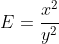Solution: putting this into the definition of elasticity, we have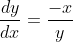,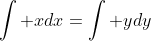,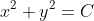, where C is a constant.

Ex.
1) Prove that a curve with elasticity 1 is hyperbolic considering the hyperbolic curve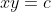.
2) Show that the elasticity of curve is independent of a linear transformation.
3) Describe the elasticity of a ellipse.
4) Describe the elasitcity of a logarithmetic function.
5) Find the curve if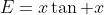6) Find the curve if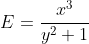7) Find the curve if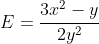(difficult!)
8) Find the maximum possible area of a triangle inscribed in a equilangular hexagon of side length 1,2,3,4,5,6 (the order of side iwth different lengths can be permuted as long as an enclosed hexagon is formed)